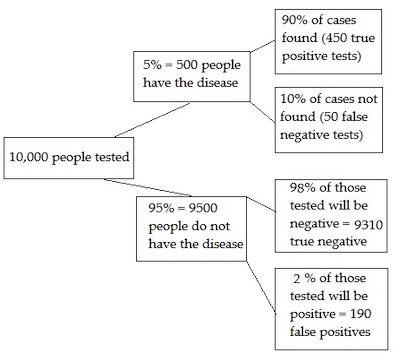### Seeing is believing!

Before you order, simply sign up for a free user account and in seconds you'll be experiencing the best in CFA exam preparation.

##### Subject 7. Multiple Tests and Interpreting Significance

A type I error is where you incorrectly reject the null hypothesis; In other words, you get a false positive. If we test a hypothesis millions of times, it can result in hundreds of thousands of false positives. The false discovery rate (FDR) is the expected proportion of type I errors.

The FDR is the expected ratio of the number of false positive classifications (false discoveries) to the total number of positive classifications (rejections of the null). The total number of rejections of the null include both the number of false positives (FP) and true positives (TP). Simply put, FDR = FP / (FP + TP).

For a more humorous (an perhaps understandable) look at the problem, take a look at XKCD's "Jelly Bean Problem."(https://xkcd.com/882) The comic shows a scientist finding a link between acne and jelly beans, when a hypothesis was tested at a 5% significance level. Although there is no link between jelly beans and acne, a significant result was found (in this case, a jelly bean caused acne) by testing multiple times. Testing 20 colors of jelly beans, 5% of the time there is 1 jelly bean that is incorrectly fingered as being the acne culprit. The implications for false discovery in hypothesis testing is that if you repeat a test enough times, you�re going to find an effect, but that effect may not actually exist.

Example

In medical testing, the false discovery rate is when you get a �positive� test result but you don�t actually have the disease.Out of 10,000 people given the test, there are 450 true positive results (box at top right) and 190 false positive results (box at bottom right) for a total of 640 positive results. Of these results, 190/640 are false positives so the false discovery rate is 30%.

If you repeat a test enough times, you will always get a number of false positives. One of the goals of multiple testing is to control the FDR: the proportion of these erroneous results. For example, you might decide that an FDR rate of more than 5% is unacceptable. Note though, that although 5% sounds reasonable, if you�re doing a lot of tests, you'll also get a large number of false positives; for 1000 tests, you could expect to get 50 false positives by chance alone. This is called the multiple testing problem, and the FDR approach is one way to control for the number of false positives.

The FDR approach adjusts the p-value for a series of tests. A p-value gives you the probability of a false positive on a single test; If you're running a large number of tests from small samples, you should use q-values instead.

• A p-value of 5% means that 5% of all tests will result in false positives.
• A q-value of 5% means that 5% of significant results will be false positives.

Although controlling for type I errors sound ideal (why not just set the threshold really low and be done with it?), Type I and Type II errors form an inverse of relationship; when one goes down, the other goes up and vice versa. By decreasing the false positives, you increase the number of false negatives - that's where there is a real effect, but you fail to detect it.

Learning Outcome Statements

f. describe how to interpret the significance of a test in the context of multiple tests;

CFA® 2022 Level I Curriculum, , Volume 1, Reading 6